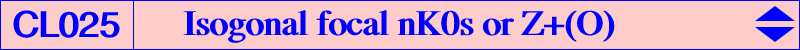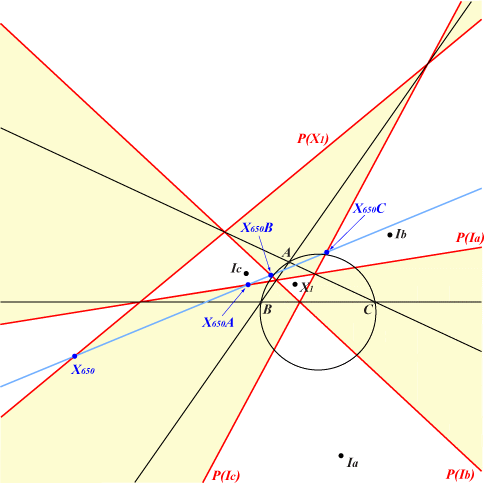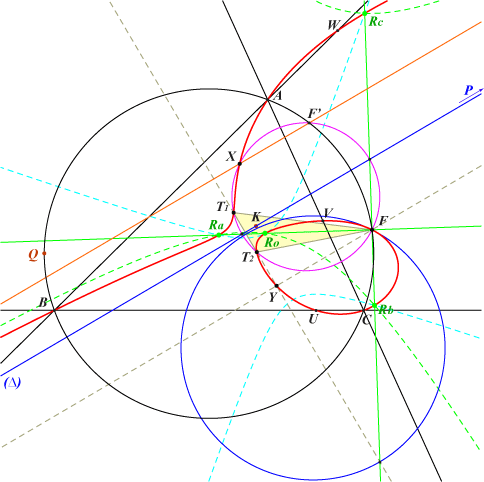We meet this type of cubics in Clark Kimberling's TCCT p. 241. See a generalization in the page P-conical cubics. For any line L through O, denote by Z+(L) the isogonal nK0 cubic with root the isogonal conjugate of the tripole of L. All these cubics are in fact focal cubics and belong to the same pencil of cubics denoted by Z+(O) containing the Brocard (second) cubic K018, the Brocard (third) cubic K019, the Pelletier strophoid K040, etc. They all pass through the (four) foci of the inscribed conic with center K = X(6) since each cubic is the locus of foci of inscribed conics centered on a line (∆) through K called the orthic line of the cubic. This line is therefore the trilinear polar of a point Q on the circumcircle. The singular focus F lies on the circumcircle and the root R on the orthic axis. The isogonal conjugate P of F is the real point at infinity. Every such cubic is also spK(P, X6), see CL055. *** The table below shows a selection of these cubics.Q R F P cubic X(98) X(230) X(110) X(523) K189 X(99) X(523) X(111) X(524) K018 X(100) X(650) X(105) X(518) K040 X(101) X(514) x X(3730) X(675) X(674) X(110) X(647) X(98) X(511) K019 X(111) X(3291) X(99) X(512) X(476) X(1637) X(842) X(542) K188 X(925) X(2501) X(3563) X(3564) K164 X(2373) X(468) X(112) X(525) K190 X(3222) X(523) x X(3552) X(699) X(698)Every isogonal focal cubic (K) = nK0(K, R) can be seen as the locus of point M such that M and its isogonal conjugate M* are conjugate points with respect to the rectangular circum-hyperbola with perspector R, a point on the orthic axis of triangle ABC. Since all these cubics are in a same pencil with basis formed by A, B, C, the circular points at infinity, the four foci of the inconic with center K, there is one and only one such cubic passing through a given point M (that does not belong to the basis) and obviously M*. In this case, • the orthic line (∆) of (K) is the line passing through the Lemoine point K and the midpoint of MM*, • the infinite point P of (∆) is the real infinite point of (K), • the singular focus F of (K) is P*, a point on the circumcircle (O) of ABC, • (K) contains the points U, V, W which are the intersections of the sidelines BC, CA, AB with the images of (∆) under the homotheties with ratio 2 and centers A, B, C respectively, • the root R of (K) is the trilinear pole of the line UVW, • the real asymptote (A) is the image of (∆) under the homothety with ratio 2, center F. *** Let (H) and (H1), (H2), (H3) be the hessian and the three prehessians of (K). Recall that one of these prehessians, say (H1), is always real and is a stelloid whose radial center is the singular focus of (K). The two other prehessians (H2), (H3) can be real (distinct or not) or imaginary conjugate. Their nature depends of the position of R with respect to the regions of the plane delimited by the trilinear polars of the in/excenters.The figure shows these regions when a > b > c. For any root R in one of the yellow surfaces, the cubic nK0(X6, R) has three real prehessians and for any R on one of the red trilinear polars, the cubic is a nodal cubic. Elsewhere, the cubic has only one real prehessian. In particular, the orthic axis meets these yellow surfaces when R lies on the segments X650-X650A and X650B-X650C where X650A, X650B, X650C are the extraversions of X650. For example, in any triangle ABC, nK0(X6, X523) = K018 has only one real prehessian, nK0(X6, X647) = K019 and nK0(X6, X2506) have three real prehessians, nK0(X6, X650) = K040 is a nodal cubic with three real prehessians but two of them coincide.The orthic line of (H1) is undetermined since (H1) is a stelloid and the four orthic lines of (K), (H), (H2), (H3) coincide. The polar conics of the infinite point P of (K) with respect to (K) and (H) are rectangular hyperbolas meeting at four (not always real) points Ro, Ra, Rb, Rc. Let us call T the diagonal triangle of the quadrilateral. Its vertices are the centers of the polar conics of P with respect to the three prehessians and one of them must be F. The two other T1, T2 are real if and only if (H2), (H3) are themselves real and, in this case, (K) is a pivotal isogonal focal cubic with respect to T whose pivot P must be the infinite point of one altitude of T, that passing through F.The circumcircle of T meets (K) again at X, the intersection of (K) with its real asymptote. X is the intersection of the polar lines of P in (K) and (H). X is also the antipode of F in the circumcircle of T and obviously the isopivot of (K). Hence the tangents at F, T1, T2 (and P) to (K) pass through X. The isogonal conjugate of X with respect to ABC is the third point Y of (K) on the line T1T2 and on the parallel at F to the orthic line. Note that the circle which is the polar conic of F has its center on the line T1T2. Naturally, the points Ro, Ra, Rb, Rc are the in/excenters of T. These points lie on the polar conic of P in (H1) which is a degenerate rectangular hyperbola with center F. ***(K) is an isoptic cubic, the locus of point M such that the segment XT1 and YT2 are seen from M under the same angle. Indeed, the pairs T1, T2 and X, Y are formed by isogonal conjugate points and these points are said to be conjugate points on the cubic (K). Recall that the midpoints of T1, T2 and X, Y lie on (∆). This can be generalized for any two points D, E on (K) and their isogonal conjugates D*, E*. The segments DE and D*E* are seen from any point M on (K) under the same angle.